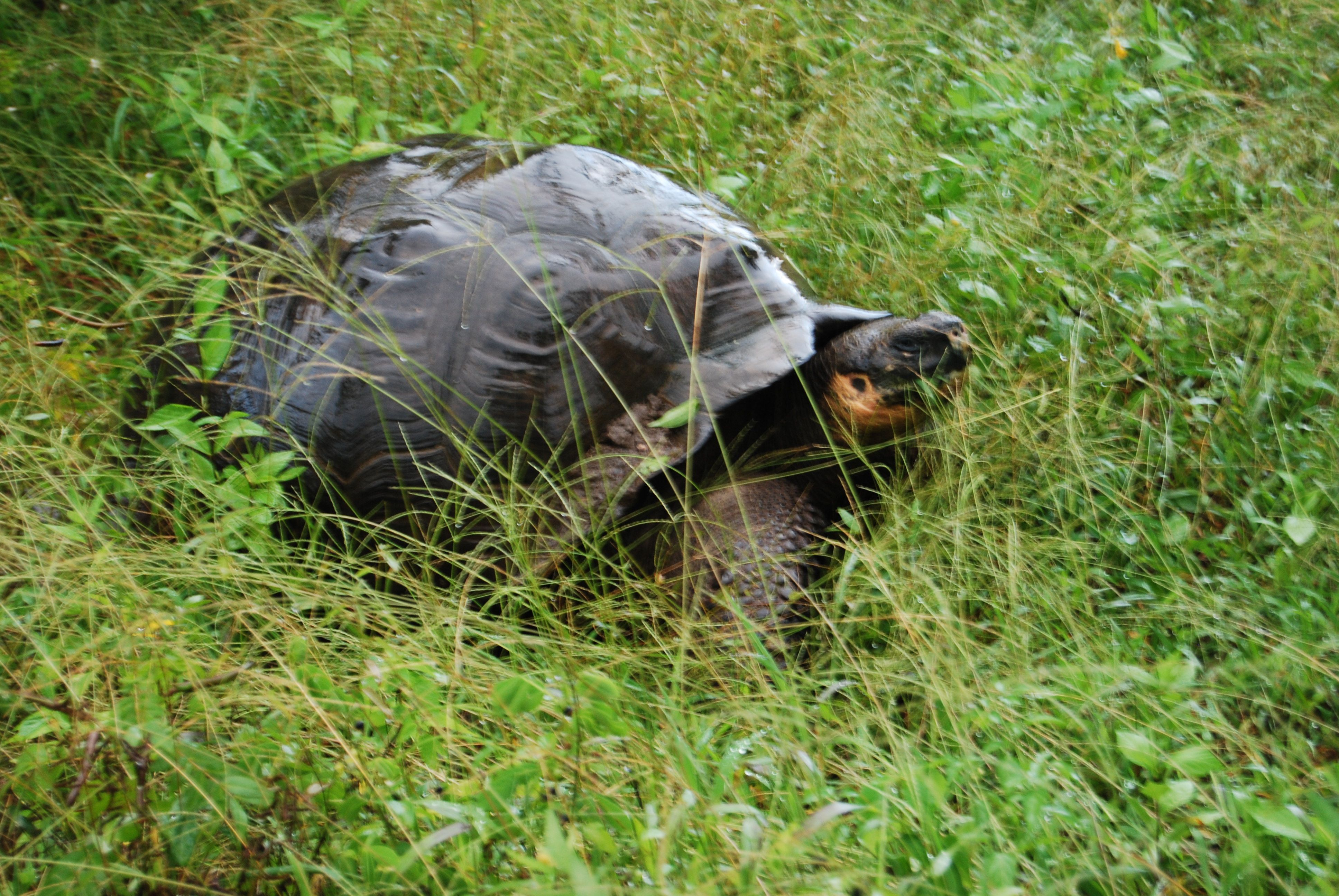# Teaching

## WFC 122  Population Dynamics and EstimationWFC 122 is designed to impart to students in the Wildlife, Fish, and Conservation Biology major an understanding of the fundamental basis of:  (1) the estimation of population parameters such as abundance and survival, and (2) the use of mathematical population models in the analysis and management of fish and wildlife populations.  The course also fulfills requirements in other majors.  The course assumes that students know the material from an introductory undergraduate course in biological statistics and two quarters of calculus.  Instruction comprises 3 hours of lecture and one three-hour laboratory per week.  Enrollment is 100 students and there are two TAs.

The material on population estimation covers definitions of standard error and confidence limits, quadrat sampling, the Leslie depletion method, Change-in-Ratio, basic mark-recapture, multiple mark-recapture, and the basic logic of computer-based estimation methods, such as the program Mark.  The emphasis is on gaining an intuitive idea of how estimation methods work, and a familiarity with the effects of assumptions not being met.

The material on population modeling includes a qualitative description of population dynamics in the three taxa of primary interest: birds, fish, and mammals, as well as some aspects of reptiles and amphibians.  The population models covered include simple exponential and logistic models, the Leslie matrix, and the three classical models used in fishery management (logistic, single-cohort and stock-recruitment).  These models are used to demonstrate concepts such as population stability and probability of extinction.  Examples of applications include age-structured models in the context of population control, population risk, and the effects of climate change.  There are also brief introductions to models with more than one species, and topics from resource economics, such as the discount rate.  The course ends with application of the concepts learned to a description of the current status of human populations.

## WFC 142  Marine Conservation Science

WFC 142 is under development.  The goal of the course is to describe what is known about how humans affect the ocean, and how we can conserve the resources we make use of from the ocean.

## WFC 222  Advanced Population Dynamics

Simply put, WFC 222 focuses on what makes populations increase and decrease on various temporal and spatial scales.  It covers most of the reasonable kinds of population models that we use to describe and analyze this variability in abundance or biomass, and most of the applications in conservation ecology.  We begin with a somewhat philosophical description of what we hope to get out of representing population models by equations, and how best to go about doing so.  We then start with the simplest models, those models with a single variable, e.g., abundance or biomass, depending on time.  We use these models to introduce most of the basic concepts involved in population behavior throughout the book, e.g, stability, persistence, etc..  We then study the behavior of models with the purest form of population dynamics, age-structured models.  These are purest in the sense that dynamics ultimately depend on interactions between various parts of a system with various time lags, and age is in units of time.  Because the size of plants and animals is often much easier to determine than age and the fact that temporal changes in growth rates often fiddle with the time delays when vital rates are size dependent, we next turn to size dependent models, i.e., models that keep track of the number of individuals at each size.  Next we turn our attention to related models that attempt to represent size structure in discrete increments with matrix models.  So far we will have assumed that all of the dynamic interactions occur at one place, so we next relax that assumption, to describe populations in which dynamic behavior varies over space, and movement is important.  Near the end of the course we make use of our accumulated knowledge to describe applications to the problems of managing population risk, managing fisheries through conventional means as well as managing biodiversity and fisheries spatially with mean protected areas.

The material is, of course, mathematical, and is intended for students of theoretical ecology, but it we also intend to build knowledge of the principles of population dynamics for ecologists in general.  The math used will go beyond calculus to matrices, partial differential equations and other topics, but we will focus on using and understanding the results of more mathematical manipulations, rather than training students to become mathematical experts in these fields.